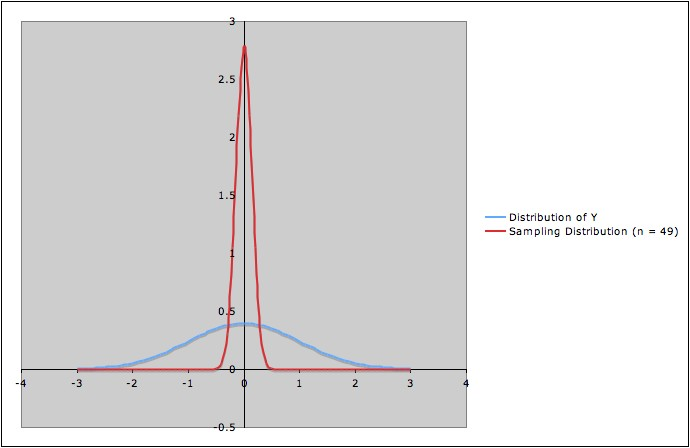COMMON MISTEAKS MISTAKES IN USING STATISTICS: Spotting and Avoiding Them

# Chart and Diagram for Confidence Interval Example

This chart and diagram may help clarify the terminology and ideas involved in understanding the concept of confidence interval.

The example: Y is a normal random variable; we are interested in a confidence interval for the mean
µ of Y.

 Population One Simple Random Sample y1, y2, ... , yn All Simple Random Samples of size n Associated Mean(s) Population mean µ, also called E(Y), or the expected value of Y, or the expectation of Y. Sample mean ȳ = (y1+ y2+ ... + yn)/n 1) Each sample has its own mean ȳ. This allows us to define a random variable Ȳn. The population for Ȳn is all simple random samples from Y. The value of Ȳn for a particular simple random sample is the sample mean ȳ for that sample. 2)  Since it is a random variable,   Ȳn also has a mean, E( Ȳn). Using the model assumptions  for this particular example, it can be proved that E( Ȳn) = µ. In other words, Y and Ȳn have the same mean as random variables. Associated Distribution Distribution of Y None Sampling Distribution (Distribution of Ȳn )

The diagram shows the distribution of Y (in blue) and the sampling distribution (distribution of  Ȳn, in red) when Y is standard normal and n = 9. Notice that both distributions have the same mean.Last updated May 7, 2012